All You Need to Know About

# SUBSTITUTE Formula in Excel

The Excel SUBSTITUTE Formula is one of the most used Excel Formulas. Learn about the different ways you can apply the SUBSTITUTE Function in Excel!

Here are the top things on what you can do with the SUBSTITUTE Formula in Excel:

## Cleaning Data with Excel’s SUBSTITUTE Formula

What does it do?

Substitutes new_text for old_text in a text string.

Formula breakdown:

=SUBSTITUTE(text, old_text, new_text, [instance num])

What it means:

=SUBSTITUTE(This cell, By this text character, To this text character, [In the 1st, 2nd…instance it occurs])

When you needed to replace a specific text in each word, and there is a pattern, Excel has just the formula for you.

The SUBSTITUTE formula in Excel can help you replace one specific text with another easily.

In our example below, we have a list of part numbers.

We want to replace the second dash  –  with the number sign  # . This formula is able to do this for us.

I explain how you can do this in this animated tutorial:

STEP 1: We need to enter the Substitute function next to the cell that we want to clean the data from:

## =SUBSTITUTE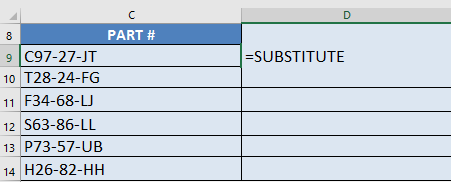STEP 2: The Substitute arguments:

## text

Which text do we want to change?

Reference the cell that contains the text or value:

## =SUBSTITUTE(C9,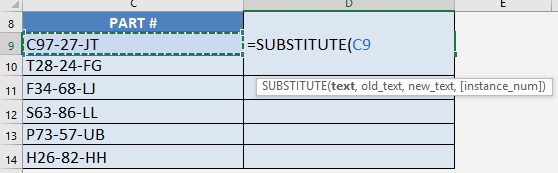## old_text

Which text / characters do we want to replace?

We want to change the dash    so type it in with double quotations:

## =SUBSTITUTE(C9, “-“,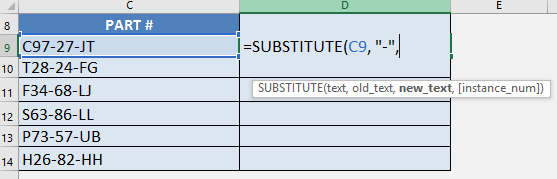## new_text

Which text / characters do we want to replace it with?

We want to change it into the number sign  #  so type it in with double quotations:

## =SUBSTITUTE(C9, “-“, “#”,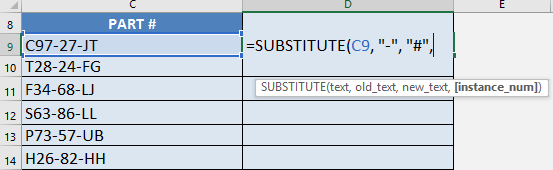## [instance num]

Which specific instance are we targeting the substitution on?

This parameter is optional. In our scenario, we want the second dash    only to be substituted. So place in the number 2:

## =SUBSTITUTE(C9, “-“, “#”, 2)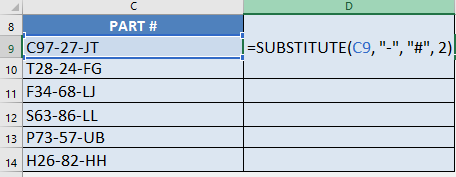STEP 3: Do the same for the rest of the cells by dragging the SUBSTITUTE formula all the way down using the left mouse button.

Note that all of the parts are now changed to your new part values: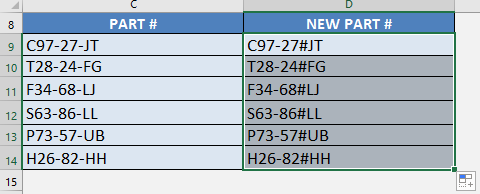## Remove Second Hyphen with Excel’s SUBSTITUTE Formula

What does it do?

Substitutes new_text for old_text in a text string.

Formula breakdown:

=SUBSTITUTE(text, old_text, new_text, [instance num])

What it means:

=SUBSTITUTE(This cell, By this text character, To this text character, [In the 1st, 2nd…instance it occurs])

There are times where I needed to remove the nth occurrence from my text.  For example I would like to remove the second hyphen in my part numbers.

Normally I would have to find the second hyphen, split the text based on the hyphen’s location, merge the remaining parts together….pfff….it’s just a headache and prone to errors!!

The SUBSTITUTE formula in Excel can actually do this for you! You might be thinking, “How can substitute remove characters?”

The secret is, we use SUBSTITUTE to replace our target character with an empty string!

In our example below, we have a list of part numbers and we want to remove the second dash.

I explain how you can do this below:

STEP 1: We need to enter the Substitute function next to the cell that we want to clean the data from:

## =SUBSTITUTE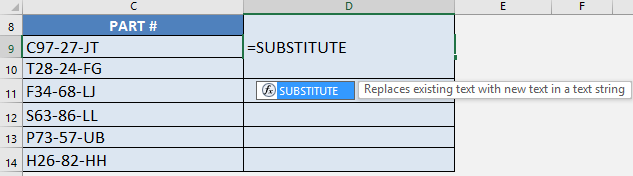STEP 2: The Substitute arguments:

## text

Which text do we want to change?

Reference the cell that contains the text or value:

## =SUBSTITUTE(C9,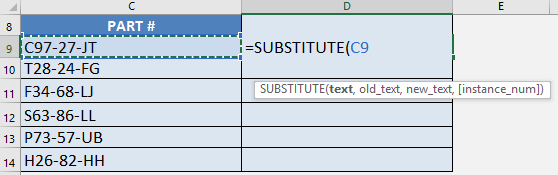## old_text

Which text / characters do we want to replace?

We want to remove the dash    so type it in with double quotations:

## =SUBSTITUTE(C9, “-“,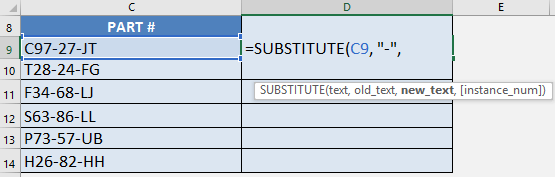## new_text

Which text / characters do we want to replace it with?

We want to remove this, so just type in a blank value:

## =SUBSTITUTE(C9, “-“, ,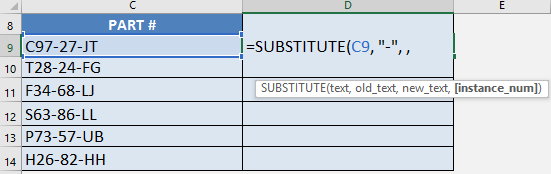## [instance num]

Which specific instance are we targeting the substitution on?

This parameter is optional.  In our scenario, we only want the second dash to be removed.  So place in the number 2 (as it is the 2nd instance the dash is located):

## =SUBSTITUTE(C9, “-“, , 2)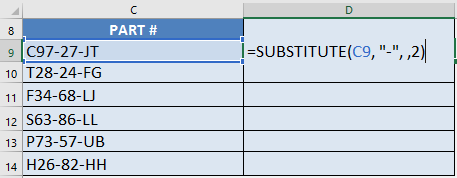STEP 3: Do the same for the rest of the cells by dragging the SUBSTITUTE formula all the way down using the left mouse button.

Note that all of the parts are now changed to your new part values: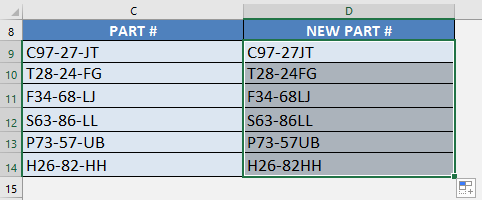## Count Text Occurrences with Excel’s SUBSTITUTE Formula

What does it do?

It replaces specific text in a text string i.e. Substitutes new text for old text in a text string.

Formula breakdown:

=SUBSTITUTE(text, old_text, new_text, [instance num])

What it means:

=SUBSTITUTE(This cell, From this TEXT character, To this TEXT character, [In the 1st, 2nd…instance it occurs])

I had a scenario where I had to count the number of occurrences of a specific character in a cell.

For example, I had to check the number of asterisks * in a cell.  Why you ask?

Well it was because the asterisks corresponded to the number of times the employee was away that year.  Who would’ve thought of putting asterisks, but there you go!

At first, I had no clue on how to do this and it was very cumbersome to do this manually!

Turns out, the SUBSTITUTE formula in Excel can be used creatively to extract the count easily!

Here’s an example:We want to return the count of asterisks here, which is 7. We will use a combination of the LEN formula and the  SUBSTITUTE formula.

We will have 3 main steps:

• Get the length of the entire string – in this case, the length of “Bradley Strickland *******” would be 26.
• Get the length of the text without the asterisks – in this case, the length of “Bradley Strickland” would be 19.
• Subtract the two lengths – this will give us 7, which is the number of asterisks.

I explain how you can do this below:

STEP 1: We need to use the SUBSTITUTE function to remove all of the asterisks from the text. We will replace the asterisk “*” with an empty string “”.

## =SUBSTITUTE(C9, “*”, “”)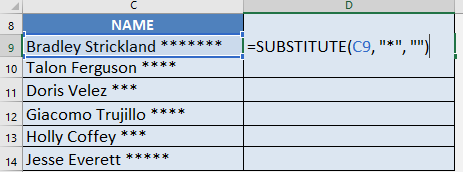You can see now that the asterisks are removed: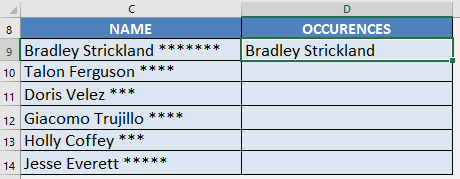STEP 2: With this result, we can get the length of the text without asterisks using the LEN function:

## =LEN(SUBSTITUTE(C9, “*”, “”))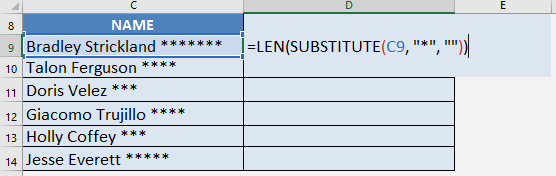The length of “Bradley Strickland” is 19: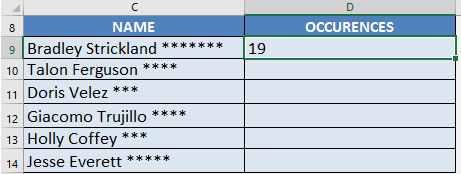STEP 3: Now here is the cool part!

Since we have the length of the text without asterisks, we now get the length of the complete text with asterisks, then we subtract them together. This will give us the number of asterisk occurrences in the text!

## =LEN(C9) – LEN(SUBSTITUTE(C9, “*”, “”))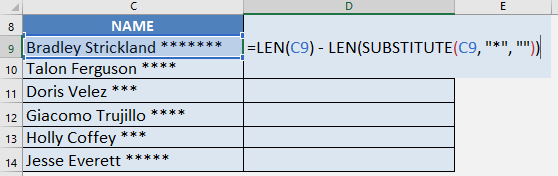This formula gives us 26 – 19, wherein 26 is the length of the whole text, while 19 is the length of the text without asterisks.

This will give us 7, which is the exact number of asterisks in the text!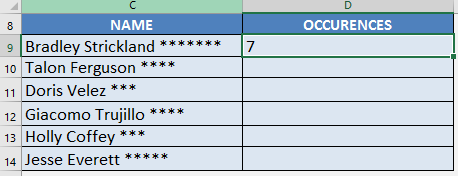STEP 4: Do the same for the rest of the cells by dragging the SUBSTITUTE formula all the way down using the left mouse button.

Note that all of the counts are automatically calculated: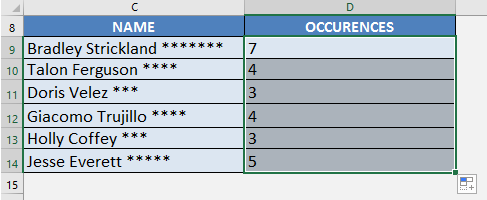Latest Tutorials

March 11, 2021

January 20, 2021

June 18, 2020

October 25, 2016

October 12, 2016

[l]
[l]
[l]
[l]
[l]
[l]
[l]
[l]
[l]
[l]
[l]
[l]
[l]
[l]
[l]
[l]
[l]
[l]
[l]
[l]
[l]
[l]
[l]
[l]
[l]
[l]
[l]
[l]
[l]
[l]